Using knowledge of planimetry for solving some systems of algebraic equations

Aim: Perfecting students' skills for transferring knowledge of planimetry (metric dependencies in a right triangle, triangle's area and cosine rule) for solving systems of quadratic equations with tree or two unknowns (9th and 10th grade).

Example 1. If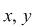and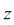are positive numbers and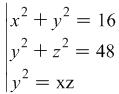then without finding the values forandfind the value of the expression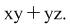Solution: If we have to solve this system it would be easy for anyone manage to findandbut in this problem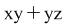is to be found. The system can be written down in the form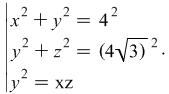Sinceandare positive, from the first equationand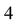can be considered using a reverse Pythagorean theorem as the lengths respectively of the legs and the hypotenuse in a right triangle ABD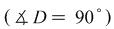(fig.1)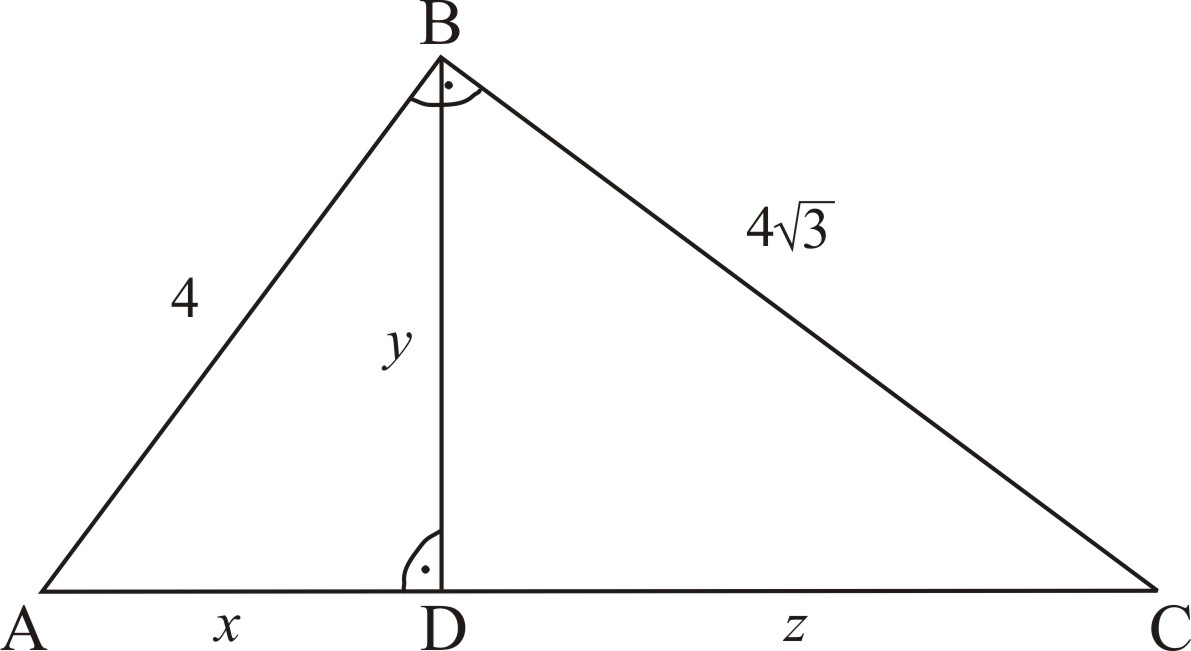Figure 1.

If we consider the second equation of the system we can reach an analogical conclusion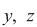и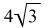are the respective lengths of the legs and hypotenuse in a triangle BCD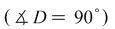(fig.1).

From the third equation we can make the conclusion that y is a number which is a geometrical average of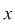and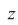, and using the reverse theorem for proportional line segments in a right triangle the consequence is that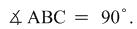Now we examine the expression, which can be represented as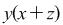. Considering figure 1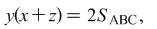but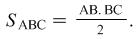In this way we find out that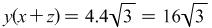i.e.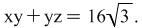For this problem the question could be to find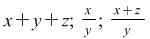.

Example 2. Ifandare positive numbers and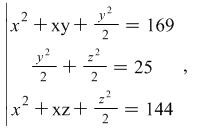then without finding values forand, calculate the expression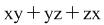.

Solution: The given system we represent in the form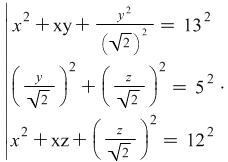Since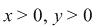and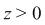, considering the second equation of the system and having in mind a reverse Pythagorean theorem the numbers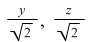and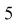are the lengths respectively of the legs and hypotenuse in a right triangle AMC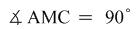(fig.2).

If we consider the first equation the numbers,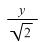and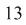are lengths of sides in a triangle AMB in which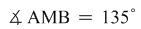and using a reverse cosine rule. Analogically,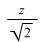and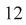are lengths of sides in a triangle BMC where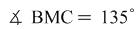( fig. 2).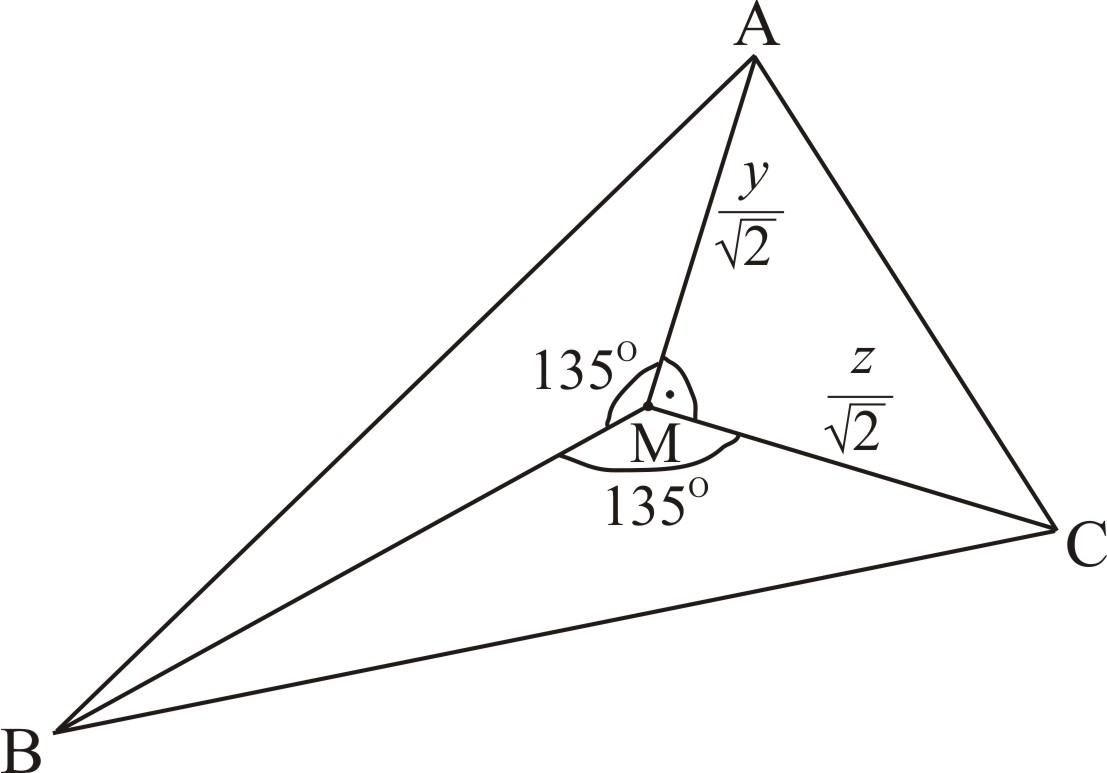Figure 2.

Since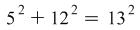, then using a reverse Pythagorean theorem we can conclude that ABC is a right triangle and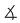ACB =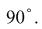We will find the areas of the following three triangles: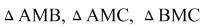using which the area of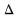ABC can be found.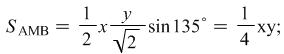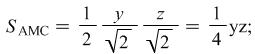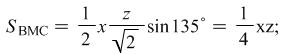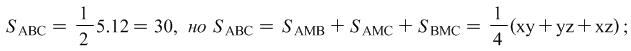This way we get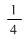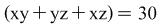, i.e.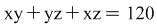.

#### For individual work

Problem 1. If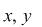andare positive numbers and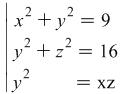then without finding the values ofandfind the value of the expressionProblem 2. Find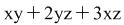, if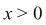,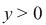,and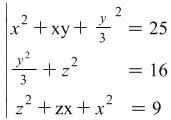Answer: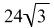.

Problem 3. If,,does the system have a solution: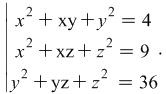Hint: If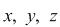are solutions to the system, than the geometric interpretation of the system is presented in figure 3. Use the inequality between the sides of a triangle i.e.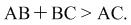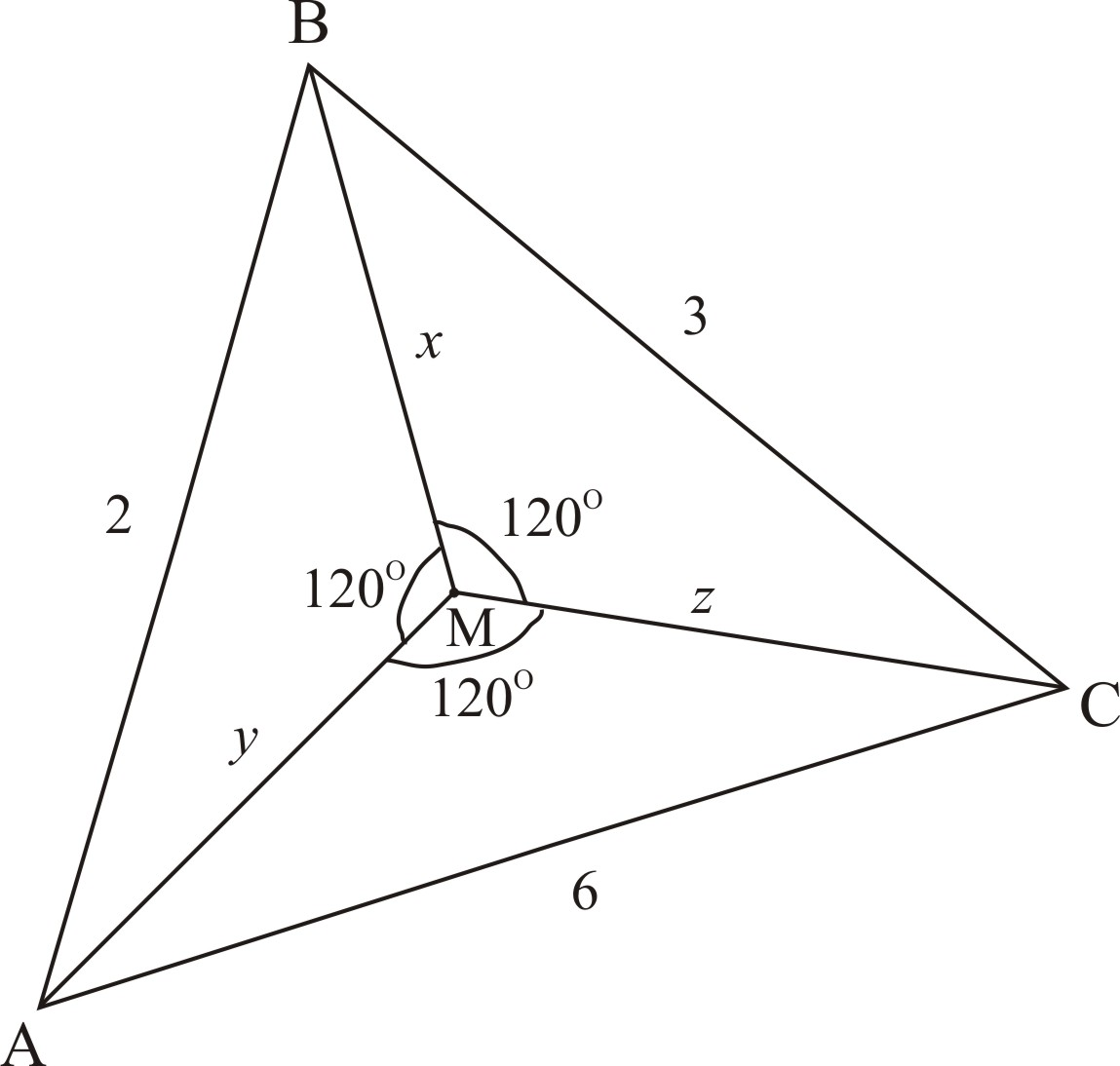Figure 3.

Problem 4. Calculate the value of the expression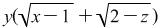, ifand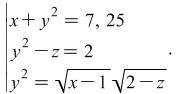Hint: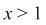and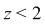, because if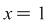and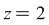, then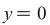. The given system we represent in the form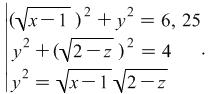Look above at the solution of problem 1.

Problem 5. Solve the system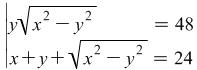.

Hint: It is not hard to prove thatand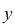are positive numbers and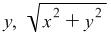and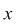are lengths of the legs and hypotenuse in a right triangle.

Answer: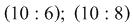.

By Rumyana Mavrova, Plovdiv university, rummav@uni-plovdiv.bg